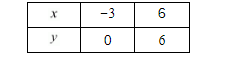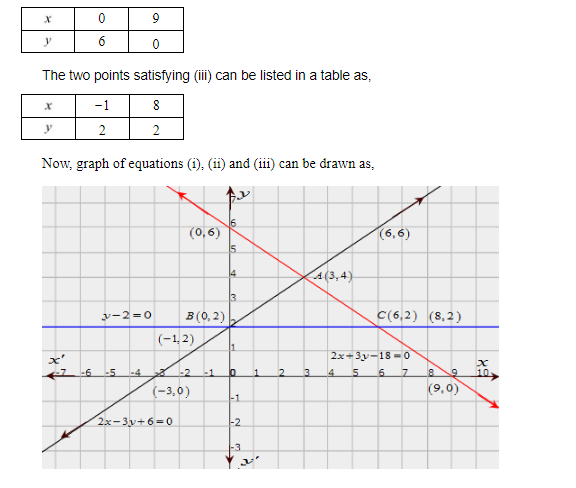# Draw the graphs of the following equations:

Question:

Draw the graphs of the following equations:

$2 x-3 y+6=0$

$2 x+3 y-18=0$

$y-2=0$

Find the vertices of the triangle so obtained. Also, find the area of the triangle.

Solution:

The given equations are

$2 x-3 y+6=0$..(i)

$2 x+3 y-18=0$..(ii)

$y-2=0$..(iii)

The two points satisfying (i) can be listed in a table as,The two points satisfying (ii) can be listed in a table as,It is seen that the coordinates of the vertices of the obtained triangle are

$A(3,4), B(0,2), C(6,2)$

Area of $\triangle A B C=\frac{1}{2} \times$ Base $\times$ height $=\frac{1}{2} \times 6 \times 2 \mathrm{sq}$ units $=6 \mathrm{sq}$ units